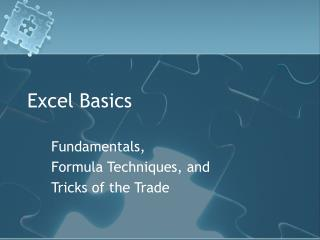DownloadDownload PresentationExcel Basics

# Excel Basics

Download Presentation## Excel Basics

- - - - - - - - - - - - - - - - - - - - - - - - - - - E N D - - - - - - - - - - - - - - - - - - - - - - - - - - -
##### Presentation Transcript

1. Excel Basics Fundamentals, Formula Techniques, and Tricks of the Trade

2. Fundamentals The basics of spreadsheet models

3. Fundamentals 1] Never enter the same information more than once. =B4

4. Fundamentals 2] Code inputs blue. When a blue input variable is changed ALL DEPENDENT values change accordingly. =B4 =B4 ? =E4 ?

5. Fundamentals 3] Name constants. =CPN

6. Go to B13 Assign names in the name box (under the toolbar). This replaces the name B13 with PRI Use [Insert] [Names…] [Define] to see and manage names used in the spreadsheet In 2007 use [Formulas] [NameManager]

7. Fundamentals 3] Name constants. Principal =CPN =RDT =PRI =SDT

8. Fundamentals 3] Name constants and arrays • Highlight • Enter the name • Shift+Ctrl+Enter =Codes

9. Fundamentals 4] Format for clarity and ease of use. vs

10. \$A\$1 A1 B1 \$A1 \$A1 A\$1 B\$1 \$A\$1 \$A\$1 A2 \$A2 A\$1 Fundamentals 5] Always line up repetitive formulas so they can be dragged across rows and down columns. *Use [F4] to toggle the lock code

11. Fundamentals 6] Train yourself to use shortcuts • Customize your toolbar • Alt+ codes • TRY Alt + I N D

12. In-Session Exercise Bond Calculator

13. Dates Nov 8 is a date Ctrl+Shift+1 and it changes to 39,758.00

14. Formats In B13 Ctrl+Shift+1 and it changes to 98.75 Ctrl+Shift+2 and it changes to 6:00PM (.75 through the day) Ctrl+Shift+3 and it changes to 7-Apr-00(Apr 7, 1900) Ctrl+Shift+4 and it changes to \$98.75 Ctrl+Shift+5 and it changes to 9875% Ctrl+Shift+6 and it changes to 9.88E+01

15. Bond Calculator

16. Bond Calculator • Compute Base Price • Note that when you use = and point to the Principal Excel uses the Name Principal rather than B3 • when you use = and point to Price Excel uses the Name PRI rather than B13

17. Functions Using Excel Functions

18. Functions • There are hundreds of built-in functions • Find them by clicking on fx • Most of the ones we use are under Financial

19. Function: COUPDAYSBS Use names See each step Description of function Use \$A\$7 which will default to 0

20. Bond Calculator Note that everything is calculatedand coded black

21. Functions • On any function click on the [fx] to open the applet.

23. Data Validation Drop Down Lists

24. Data Validation B7 Set to accept inputs from B19:B21only

25. Drop Down Lists in Excel • From B7 use Data/ Validation • Choose List and specify \$B\$19:\$B\$21 List Dropdown \$B\$19:\$B\$21

26. Drop Down Lists The drop down forces the user to choose from a list of inputs

27. Lookup Finding specific information

28. Lookup Basis for COUP functions • In A7=VLOOKUP(B7,Codes,2,FALSE) Exact match only Find the value in B7 in the 1st column of… The array we named Codes Get the corresponding value in column 2 =Codes

29. Lookup • Choose a different Bond type and watch the code change. • vLookup • Looks up in the first column of an array and matches the row • hLookup • Looks up in the first row of an array and matches the column

30. If Excel conditions

31. =IF(Condition, True, False) =IF(A7=1,"Treasury","Not a Treasury") =IF(TEXT(RDT,"dd")="15", "matures on the 15th", "doesn't mature on the 15th") Day part of SDT

32. =IF(Condition, True, False) =IF(A7=1, IF(TEXT(B5,"dd")="15", "", "Treasuries mature on the 15th"), "") You can embed up to 7 if statements in one cell

33. Tables Sensitivity Analysis

34. Data Tables • Change one input in a model and calculate what various outputs would be • Change two inputs in a model and calculate what one output would be

35. {=Table()} =E16 Cell where the Nominal Yield is calculated Change the price quote (from 60 to 135) =E17 Cell where income Yield is calculated =E18 Cell where YTM is calculated

36. {=Table()} • Highlight the table area • The first column is the list of prices • The top row is the list of outputs

37. \$B\$13 {=Table()} 2.Use [Data][Table] 3.Indicate that the first column in the area is to replace cell B13 4.[OK]

38. {=Table()} Test the table array by changing the input n and comparing the results with the table

39. Graphs When a picture is worth a thousand words

40. Graphs • What are you trying to communicate? • How do you best communicate it?

41. Combined Chart Monarch Price of a quarter of wheat Wage of a good mechanic

42. Florence Nightingale Deaths from preventable disease Deaths from wounds Deaths from all other causes

43. Napoleon's March on Russia 1812-1813

44. Graphs • Chart as an xy scatter chart • exchange the x and y axes • Yield shouldbe the x axis • Price shouldbe the y axis • Use right click/source data

45. Chart Area The Chart Area is the frame in which the chart is held. Set the chart font to the same as the spreadsheet and de-click autoscale

46. Plot Area The Plot Area is the chart space. Highlight the grey and delete; highlight the gridlines and delete or reformat to make them less obnoxious.Expand the Plot Area to enlarge the graph within the frame

47. Axes Edit Chart Options to add Titles Format Axes

48. Format Data Series Format Data point to add label Color nominal yield white to hide it

49. Format Data Series Change to Income Alt+Enter Yield The label Changes as well

50. Conditional Formatting Font color, highlights, etc